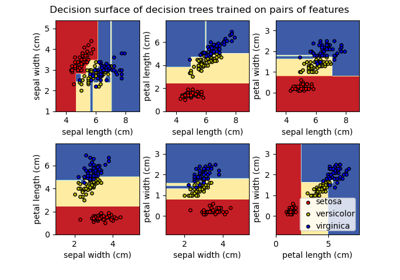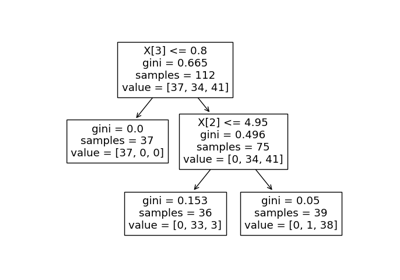# sklearn.tree.plot_tree¶

sklearn.tree.plot_tree(decision_tree, *, max_depth=None, feature_names=None, class_names=None, label='all', filled=False, impurity=True, node_ids=False, proportion=False, rounded=False, precision=3, ax=None, fontsize=None)[source]

Plot a decision tree.

The sample counts that are shown are weighted with any sample_weights that might be present.

The visualization is fit automatically to the size of the axis. Use the figsize or dpi arguments of plt.figure to control the size of the rendering.

Read more in the User Guide.

New in version 0.21.

Parameters:
decision_treedecision tree regressor or classifier

The decision tree to be plotted.

max_depthint, default=None

The maximum depth of the representation. If None, the tree is fully generated.

feature_nameslist of strings, default=None

Names of each of the features. If None, generic names will be used (“X”, “X”, …).

class_nameslist of str or bool, default=None

Names of each of the target classes in ascending numerical order. Only relevant for classification and not supported for multi-output. If True, shows a symbolic representation of the class name.

label{‘all’, ‘root’, ‘none’}, default=’all’

Whether to show informative labels for impurity, etc. Options include ‘all’ to show at every node, ‘root’ to show only at the top root node, or ‘none’ to not show at any node.

filledbool, default=False

When set to True, paint nodes to indicate majority class for classification, extremity of values for regression, or purity of node for multi-output.

impuritybool, default=True

When set to True, show the impurity at each node.

node_idsbool, default=False

When set to True, show the ID number on each node.

proportionbool, default=False

When set to True, change the display of ‘values’ and/or ‘samples’ to be proportions and percentages respectively.

roundedbool, default=False

When set to True, draw node boxes with rounded corners and use Helvetica fonts instead of Times-Roman.

precisionint, default=3

Number of digits of precision for floating point in the values of impurity, threshold and value attributes of each node.

axmatplotlib axis, default=None

Axes to plot to. If None, use current axis. Any previous content is cleared.

fontsizeint, default=None

Size of text font. If None, determined automatically to fit figure.

Returns:
annotationslist of artists

List containing the artists for the annotation boxes making up the tree.

Examples

>>> from sklearn.datasets import load_iris
>>> from sklearn import tree

>>> clf = tree.DecisionTreeClassifier(random_state=0)

>>> clf = clf.fit(iris.data, iris.target)
>>> tree.plot_tree(clf)
[...]


## Examples using sklearn.tree.plot_tree¶Plot the decision surface of decision trees trained on the iris dataset

Plot the decision surface of decision trees trained on the iris datasetUnderstanding the decision tree structure

Understanding the decision tree structure